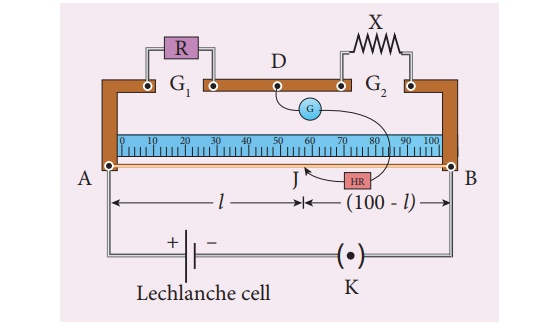Home | | Physics 12th Std | Specific Resistance of the Material of the Coil Using Metre Bridge

# Specific Resistance of the Material of the Coil Using Metre Bridge

To determine the specific resistance of the material of the given coil using metre bridge.

SPECIFIC RESISTANCE OF THE MATERIAL OF THE COIL USING METRE BRIDGE

## AIM

To determine the specific resistance of the material of the given coil using metre bridge.

## APPARATUS REQUIRED

Meter bridge, galvanometer, key, resistance box, connecting wires, Lechlanche cell, jockey and high resistance.

## FORMULAwhere,

X ŌåÆ Resistance of the given coil (Ōä”)

R ŌåÆ Known resistance (Ōä”)

L ŌåÆ Length of the coil (m)

r ŌåÆ Radius of the wire (m)

## CIRCUIT DIAGRAM## PROCEDURE

┬Ę        A resistance box R is connected in the left gap and the unknown resistance X in the right gap.

┬Ę        A Lechlanche cell is connected across the wire of length 1 m through a key.

┬Ę        A sensitive galvanometer G is connected between the central strip and the jockey through a high resistance (HR).

┬Ę        With a suitable resistance included in the resistance box, the circuit is switched on.

┬Ę        To check the circuit connections, the jockey is pressed near one end of the wire, say A.

┬Ę        The galvanometer will show deflection in one direction. When the jockey is pressed near the other end of the wire B, the galvanometer will show deflection in the opposite directions. This ensures that the circuit connections are correct.

┬Ę        By moving the jockey over the wire, the point on the wire at which the galvanometer shows null deflection i.e., balancing point J is found.

┬Ę        The balancing length AJ = l is noted.

┬Ę        The unknown resistance X1 is found using the formula X1 = R (100 ŌłÆl) / l

┬Ę        The experiment is repeated for different values of R.

┬Ę        The same procedure is repeated after interchanging R and X.

┬Ę        The unknown resistance X2 is found using the formula X2 = Rl / (100 ŌłÆl)

┬Ę        The experiment is repeated for same values of R as before.

┬Ę        The resistance of the given coil is found from the mean value of X1 and X2.

┬Ę        The radius of the wire r is found using screw gauge.

┬Ę        The length of the coil L is measured using meter scale.

┬Ę        From the values of X, r and L, the specific resistance of the material of the wire is determined.

## OBSERVATION

length of the coil L = ____________________________________ cm.

Table 1 To find the resistance of the given coilTable 2 To find the radius of the wire

Zero error =

Zero correction =## RESULT

The specific resistance of the material of the given coil = _____________________(╬®m)

### Note:

i. To check the circuit connections:

The meter bridge wire is touched near one end (say, end A) with jockey, galvanometer shows a deflection in any one direction. Now the other end (say, end B) is touched. If the galvanometer shows a deflection in the opposite direction, then the circuit connections are correct.

ii. The usage of high resistance (HR):

The galvanometer is a very sensitive device. If any high current flows through the galvanom-eter, its coil gets damaged. Therefore in order to protect the galvanometer, a high resistance (HR) is used. When HR is connected in series with the galvanometer, the current through it is reduced so that the galvanometer is protected. But the balancing length is not accurate.

iii. To find the accurate balancing length:

The HR is first included in the circuit (that is, the plug key in HR is removed), the approx-imate balancing length is found. Now HR is excluded in the circuit (that is, the plug key in HR is closed), then the accurate balancing length is found.

Tags : Physics Practical Experiment , 12th Physics : Practical
Study Material, Lecturing Notes, Assignment, Reference, Wiki description explanation, brief detail
12th Physics : Practical : Specific Resistance of the Material of the Coil Using Metre Bridge | Physics Practical Experiment